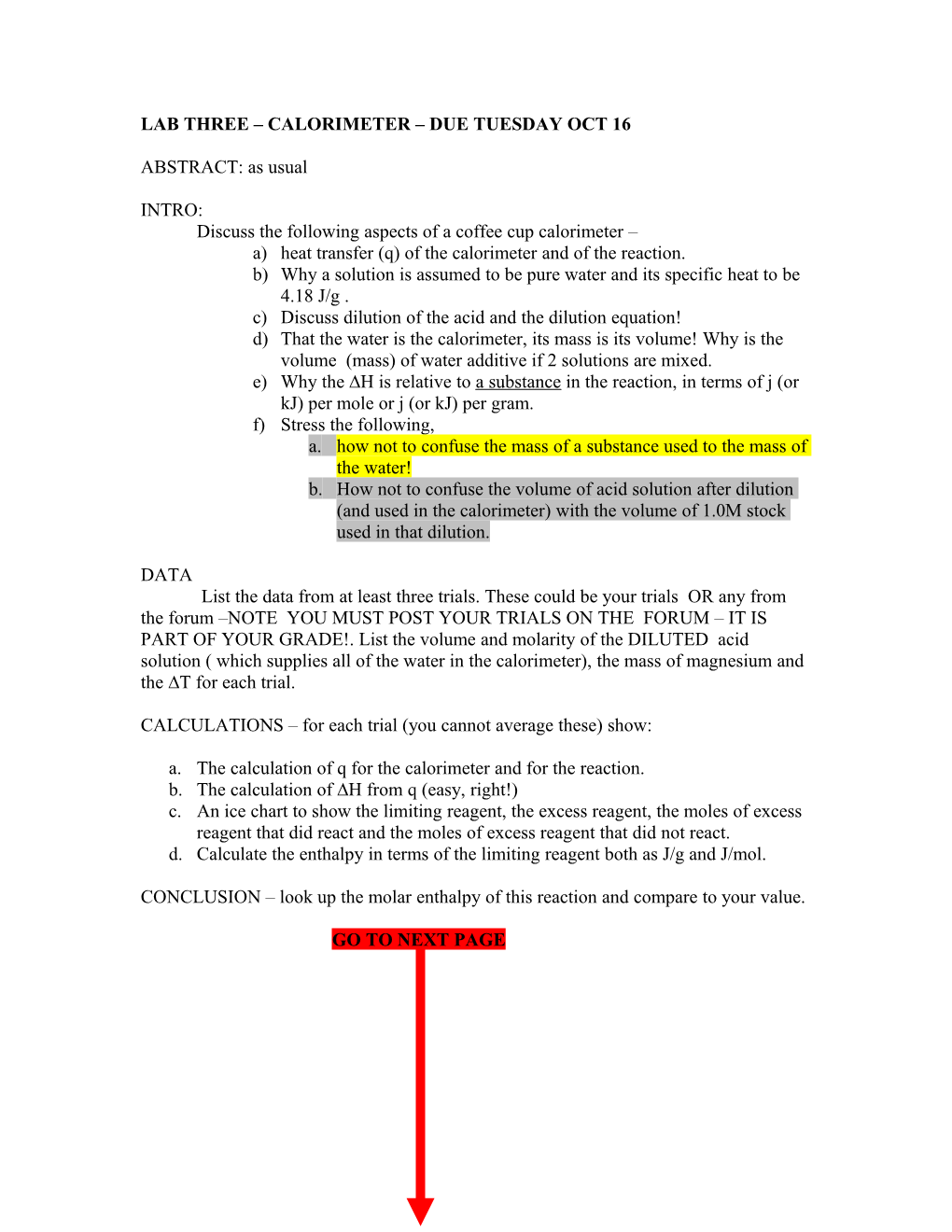# Lab Three Calorimeter Due Tuesday Oct 16LAB THREE – CALORIMETER – DUE TUESDAY OCT 16

ABSTRACT: as usual

INTRO:

Discuss the following aspects of a coffee cup calorimeter –

a)heat transfer (q) of the calorimeter and of the reaction.

b)Why a solution is assumed to be pure water and its specific heat to be 4.18 J/g .

c)Discuss dilution of the acid and the dilution equation!

d)That the water is the calorimeter, its mass is its volume! Why is the volume (mass) of water additive if 2 solutions are mixed.

e)Why the ∆H is relative to a substance in the reaction, in terms of j (or kJ) per mole or j (or kJ) per gram.

f)Stress the following,

1. how not to confuse the mass of a substance used to the mass of the water!
2. How not to confuse the volume of acid solution after dilution (and used in the calorimeter) with the volume of 1.0M stock used in that dilution.

DATA

List the data from at least three trials. These could be your trials OR any from the forum –NOTE YOU MUST POST YOUR TRIALS ON THE FORUM – IT IS PART OF YOUR GRADE!. List the volume and molarity of the DILUTED acid solution ( which supplies all of the water in the calorimeter), the mass of magnesium and the ∆T for each trial.

CALCULATIONS – for each trial (you cannot average these) show:

1. The calculation of q for the calorimeter and for the reaction.
2. The calculation of ∆H from q (easy, right!)
3. An ice chart to show the limiting reagent, the excess reagent, the moles of excess reagent that did react and the moles of excess reagent that did not react.
4. Calculate the enthalpy in terms of the limiting reagent both as J/g and J/mol.

CONCLUSION – look up the molar enthalpy of this reaction and compare to your value.

GO TO NEXT PAGE

LAB TWO: Determination of Cl- by AgNO3 titration.(Due Tuesday OCT 16)

ABSTRACT – as usual.

INTRODUCTION:

1. Discuss titrations (use chapter four).
2. Discuss concentration of solutions and molarity and molarity to mole conversions.
3. Discuss the role of solubility rules in metathesis, find five example reactions and explain them in terms of the solubility of the salts.
4. Write NET IONIC REACTIONS FOR THE FIVE REACTIONS you find.

Data:

For EACH TRIAL, list the volume of silver nitrate used and the mass of the salt sample you titrated (should be the same for each as you made one solution and divided it!). List any observations.

CALCULATIONS:

-YOU MAY AVERAGE YOUR VOLMUES AgNO3for this.

1. Calculate moles of silver nitrate dispensed.
2. Calculate miles of Cl- present.
3. Calculate % of Cl- in NaCl
4. Calculate the theoretical % of Cl- in NaCl.
5. Staple you PRACTICAL to the lab when I return them (Monday should be!)